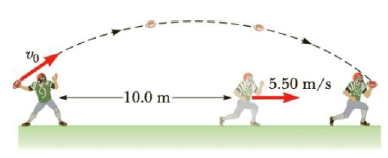Chapter 3, Problem 58AP

Chapter
Section
Textbook Problem

A football receiver running straight downfield at 5.50 m/s is 10.0 m in front of the quarterback when a pass is thrown downfield at 25.0° above the horizon (Fig. P3.58). If the receiver never changes speed and the ball is caught at the same height from which it was thrown, find (a) the football’s initial speed, (b) the amount of time the football spends in the air, and (c) the distance between the quarterback and the receiver when the catch is made.Figure P3.58

(a)

To determine
The initial speed of the football.

Explanation

The horizontal displacement of the ball is,

xball=v02sin2θg

Here,

v0 is the initial velocity

θ is the launch angle

g is the acceleration due to gravity

When the receiver catches the ball, the position of the receiver is,

xR=xR0+vRt

Here,

xR0 the initial position of the receiver

vR is the velocity of the receiver

t is the time taken

As the displacement is equal to the position of the receiver,

xball=xRv02sin2θg=xR0+vRt

Substitute t=2v0sinθg for t .

v02sin2θg=xR0+vR(2v0sinθg)(sin2θ

(b)

To determine
The amount of time the football spends in air.

(c)

To determine
The distance between the quarterback and the receiver when the catch is made.

Still sussing out bartleby?

Check out a sample textbook solution.

See a sample solution

The Solution to Your Study Problems

Bartleby provides explanations to thousands of textbook problems written by our experts, many with advanced degrees!

Get Started